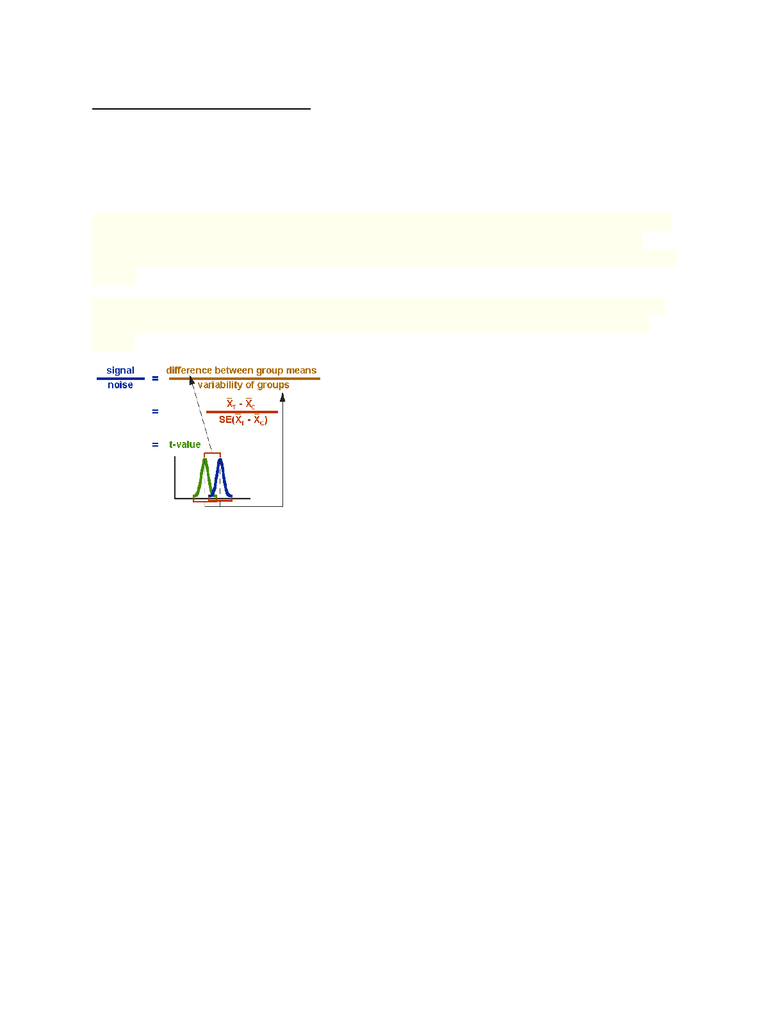# ARH312Y1 Lecture Notes - Fume Hood, Punnett Square, Statistical Hypothesis Testing

57 views3 pagesARH312 Week 1- September 13th
Drennan Statistics for Archaeologists (can be found at Robarts and online)
Fletcher and Lock- Digging Numbers: Elementary Stats for Archaeologists (found at Robarts)
Free Statistical program called PAST http://folk.uio.no/ohammer/past
The t-test assesses whether the means of two groups are statistically different from each other.
This analysis is appropriate whenever you want to compare the means of two groups, and
especially appropriate as the analysis for the posttest-only two-group randomized experimental
design.
The formula for the t-test is a ratio. The top part of the ratio is just the difference between the
two means or averages. The bottom part is a measure of the variability or dispersion of the
scores.
Chi-square is a statistical test commonly used to compare observed data with data we would
expect to obtain according to a specific hypothesis.
The formula for calculating chi-square ( 2) is:
2= (o-e)2/e
That is, chi-square is the sum of the squared difference between observed (o) and the expected
(e) data (or the deviation, d), divided by the expected data in all possible categories.
For example, suppose that a cross between two pea plants yields a population of 880 plants, 639
with green seeds and 241 with yellow seeds. You are asked to propose the genotypes of the
parents. Your hypothesis is that the allele for green is dominant to the allele for yellow and that
the parent plants were both heterozygous for this trait. If your hypothesis is true, then the
predicted ratio of offspring from this cross would be 3:1 (based on Mendel's laws) as predicted
from the results of the Punnett square (Figure B. 1).
How to write labs:
1. Intro to the problem
2. State the hypothesis
3. Materials
Unlock document

This preview shows page 1 of the document.
Unlock all 3 pages and 3 million more documents.